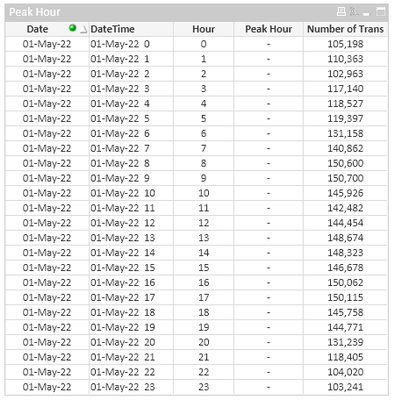Announcements
The way to achieve your own success is the willingness to help somebody else. Go for it!
cancel
Showing results for
Did you mean:Creator

## Finding Peak Hour & Peak Transaction in a day

I have the table below. In a separate table, I need to have the Date, Peak Hour, and Peak Transaction (Peak Hour is the hour with the highest transaction.  I tried the expression below and it came back with an - . It says expression OK, but nothing populates.

max({<DateTime={">=\$(=timestamp(only(Date) + only(Hour)/24 ))<\$(=timestamp(only(Date) + (only(Hour)+1)/24 ))"}>} sum(Trans). I know the sum is part of the issue, but the table below is summing all the transactions in the hour. The Number of Trans column is an expression that is sum(Trans)

** the table I attached is a summary table. The data is thousands of lines. For example, 01-May-22, at hour 0 will have a thousand transactions that total 105,198

01-May-22, 0,1500

01-May-22, 0,2900

01-May-22, 0,6257

01-May-22, 0,574This is what I want the end resulting table to look like:

 Date Peak Hour Peak Trans 01-May-22 9 150,700 02-May-22 14 172,940

Thank you,

Labels (3)

• ### Scripting

1 Solution

Accepted SolutionsCreator
Author

Here is the solution I figured out.  I had made it way too complicated (overthinking the expression). Two parts

Find peak hour:

FirstSortedValue( Hour, -aggr( sum(Trans), DateTime, Hour))

Find peak transaction:

max(aggr(sum(Trans), DateTime, Hour))

5 RepliesSpecialist II

Hi @mp802377,

Is it possible to share the sample data?

Regards,
Abhijit
keep Qliking...
Help users find answers! Don't forget to mark a solution that worked for you!Creator
AuthorSpecialist II

Regards,
Abhijit
keep Qliking...
Help users find answers! Don't forget to mark a solution that worked for you!Creator
Author

It isn't resolved. I added a text file that contains sample data. I still have the issue. When I tried max({<DateTime={">=\$(=timestamp(only(Date) + only(Hour)/24 ))<\$(=timestamp(only(Date) + (only(Hour)+1)/24 ))"}>} sum(Trans)) that didn't work. I tried max({<DateTime={">=\$(=timestamp(only(Date) + only(Hour)/24 ))<\$(=timestamp(only(Date) + (only(Hour)+1)/24 ))"}>} Trans) and that didn't work eitherCreator
Author

Here is the solution I figured out.  I had made it way too complicated (overthinking the expression). Two parts

Find peak hour:

FirstSortedValue( Hour, -aggr( sum(Trans), DateTime, Hour))

Find peak transaction:

max(aggr(sum(Trans), DateTime, Hour))Community Browser